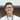# Paint can for room

March 07, 2021

This may or may not be the optimal solution, DO NOT blindly memorize my solutions

From Cassidy’s weekly newsletter.

Target audience: beginners in js (please feedback!)

# This week’s question:

Given a room size, and the square footage a paint can can cover, return how many cans of paint you need to buy to paint a room. Assume the room has four walls. If you’d like to expand this, you can add doors, windows, or any other room features that might make the problem interesting to solve.

## Example:

room = { length: 12, width: 10, height: 9 }
canCoverage = 200
$numberOfCans(room, canCoverage)$ 2 // (12x9x2)+(10x9x2) = 396, so two cans will cover it

This question is logically fairly simple, in fact most of the solution is already given in the example!

const calcCanCoverage = (length, width, height, canCoverage) =>
Math.ceil((length * height * 2 + width * height * 2) / canCoverage);

console.log(calcCanCoverage(12, 10, 9, 200));

Now the input isn’t really in that format, so let’s find some way to extract that information.

const room = { length: 12, width: 10, height: 9 };
const canCoverage = 200;

const calcCanCoverage = (length, width, height, canCoverage) =>
Math.ceil((length * height * 2 + width * height * 2) / canCoverage);

const numberOfCans = (room, canCoverage) => {
const { length, width, height } = room;
return calcCanCoverage(length, width, height, canCoverage);
};

console.log(numberOfCans(room, canCoverage));

And that’s the whole thing solved! However, since this is not much of an article, let’s attempt some input management. One of the things we want to handle is if room is not an object that has our length width or height. Here we can think of using some value to show “error”. Logically thinking, we can use either -1 or null as the error response. In this case, I’ll use -1.

const numberOfCans = (room, canCoverage) => {
const { length, width, height } = room;
if (!(length && width && height)) {
return -1;
}
return calcCanCoverage(length, width, height, canCoverage);
};

This will handle cases where length, width and height are 0, any one of those is NaN (if room is not an object) and also if any one of those is null or undefined (if room is empty object).

We should also handle if length, width and height is a number. let’s type-cast in our input validation

const numberOfCans = (room, canCoverage) => {
const { length, width, height } = room;
if (!(Number(length) && Number(width) && Number(height))) {
return -1;
}
return calcCanCoverage(length, width, height, canCoverage);
};

This will allow '12' to be type-casted into a number,'12a' will be NaN. Booleans will be converted into 1 or 0.

If we want to add another check right now, it would be quite verbose to check everything again, so let’s use Object.values on the room object, so that we get all the values, and an every function to test every integer.

const numberOfCans = (room, canCoverage) => {
const { length, width, height } = room;
if (!Object.values(room).every((val) => Number(val))) {
return -1;
}
return calcCanCoverage(length, width, height, canCoverage);
};

This introduces a new bug in that the validation will pass if room is an empty object. We could check that length width and height exists in the object, but I would just check if room is an object and has values.

const numberOfCans = (room, canCoverage) => {
const { length, width, height } = room;
if (
!room ||
Object.keys(room).length === 0 ||
!Object.values(room).every((val) => Number(val))
) {
return -1;
}
return calcCanCoverage(length, width, height, canCoverage);
};

Now the last thing I can think of is to check if the room dimensions are positive, so let’s add that in the every function

const numberOfCans = (room, canCoverage) => {
const { length, width, height } = room;
if (
!room ||
Object.keys(room).length === 0 ||
!Object.values(room).every((val) => Number(val) > 0)
) {
return -1;
}
return calcCanCoverage(length, width, height, canCoverage);
};

So that’s our validation! There’s probably some things I missed, but we’ve made our solution a little bit more “safe”. If we want to go on to write libraries, and tools that are used by many people we should practice input validation where necessary. What other things did I leave out? You can tweet @ me or respond to the dev.to article!Ten Zhi Yang is a dev in Singapore. Disagree with me? @ me on twitter. Like my post? @ me on twitter too!